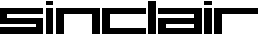ZX SPECTRUM Introduction3. Numbers, letters and the computer as a calculatorWe have already seen how to tell the computer to print letters and graphics on the screen using PRINT.  We have also seen that ENTER has to be used to tell the computer to execute the command just typed in. From now on we will not use ENTER in the manual each time a command is used, but assume  that you will key it at the end of the line automatically.Numbers can be handled by the computer more easily than letters. In the previous chapter we hinted at this by explaining that the computer expects a number after PRINT if quotations marks are not used.So if we keyPRINT 2the number 2 will appear on the screen.It is possible to mix letters and numbers:PRINT 2.öABC"Notice that there is a gap on the screen between the 2 and ABCNow keyPRINT 2;öABCöand thenPRINT 2 ōABCöUsing a comma between the items after PRINT spaces them out by 16 columns, using a semi-colon eaves no spaces and using nothing gives an errorPRINT can also be used with the mathematical functions on the keyboard. In fact the ZX Spectrum can be used as an electronic calculator.For example:PRINT 2+2The answer appears at the top of the screen. Compare this with:PRINT ō2+2"It is possible to combine these to give something more useful.  TryPRINT ō2+2=";2+2Try some other kinds of arithmetic as well:PRINT 3-2PRINT 4/5PRINT 12*2The * Is used as a multiplication sign instead of X to avoid getting it confused with the letter x; and / is used for the division sign.Experiment with lots of different calculations.  If you like you can use negative numbers or numbers with decimal points in.If you do enough to use up the 22 lines of the top par of the screen, then you will notice something rather interesting happening; it will all move up one line and the top line will be lost. This is called scrolling.Calculations are not always performed in the order you might expect. As an example, tryPRINT 2+3*5You might expect this to take 2, add 3 giving 5, and then multiply by 5 giving 25; however this is not the case. Multiplications - and divisions as well - are performed before additions and subtractions so the expression æ2+3*5Æ means  take 3 and multiply it by 5, giving 15; and then add that to 2 giving 17'. 17 should be the answer displayed on the screen.Because multiplications and divisions are done first we say that they have higher priority than addition and subtraction. Relative to each other, multiplication and division have the same priority, which means that the multiplications and divisions are done in order from left to right. When they are dealt with we are left with the additions and subtractions - these again have the same priority as each other so we do them in order from left to right.Let us see How the computer would work out:PRINT 20-2*9+4/2*3i     20-2*9+4/2*3  First we do the multiplications and divisions in order from left to rightii    20-18+4/2*3  iii   20-18+2*3iv   20-18+6}  and then the additions and subtractionsv    2+6}vi   8}Although all you really need to know is whether one operation has a higher or lower priority than another, the computer does this by having a number between 1 and 16 to represent the priority of each operation: * and / have priority 8, and + and  have priority 6.This order of calculation is absolutely rigid, hut you can circumvent it by using brackets, anything in brackets is evaluated first and then treated as a single number, so thatPRINT 3*2+2gives the answer 6+2=8 butPRINT 3*(2+2)gives the answer 3*4=12It is sometimes useful to give the computer expressions such as this because whenever the computer is expecting a number from you, you can give it an expression instead and it will work out the answer. The exceptions to this rule are so few that they will be stated explicitly in every case.You can write numbers with decimal points (use the full stop}, and you can also use scientific notation - as is quite common on pocket calculators. In this after an ordinary number (with or without a decimal point) you write an exponent part consisting of the letter e then maybe -, and then a number. The exponent part shifts the decimal point along to the right (or left, for a negative exponent) thus multiplying (or dividing) the original number by lea few times.  For instance2.34e0=2.342.34e3=23402.34e-2=0.0234 and so on(Try printing these out on the computer.) This is one of the few cases where you can't replace a number by an expression: for instance, you cannot write(1.34+1)e(6/2).You can also have expressions whose values are not numbers, but strings of letters. You have seen the simplest form of this many times, the string of letters written down with double quotes around it. This is rather analogous to the simplest form of numeric expression, which is just a number written down on its own. What you have not yet seen is the use of + with strings (but not -, * or /, so there is no problem with priorities here).  Adding strings together just joins them together one after the other: so tryPRINT "jers"+"ey cow"You can add together as many strings as you like in a single expression, and if you want, you can even use brackets.[C]